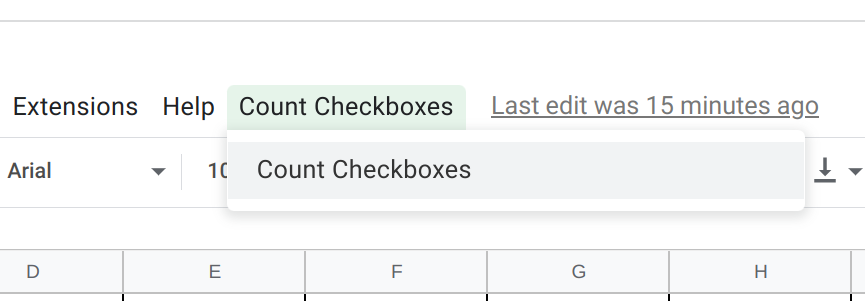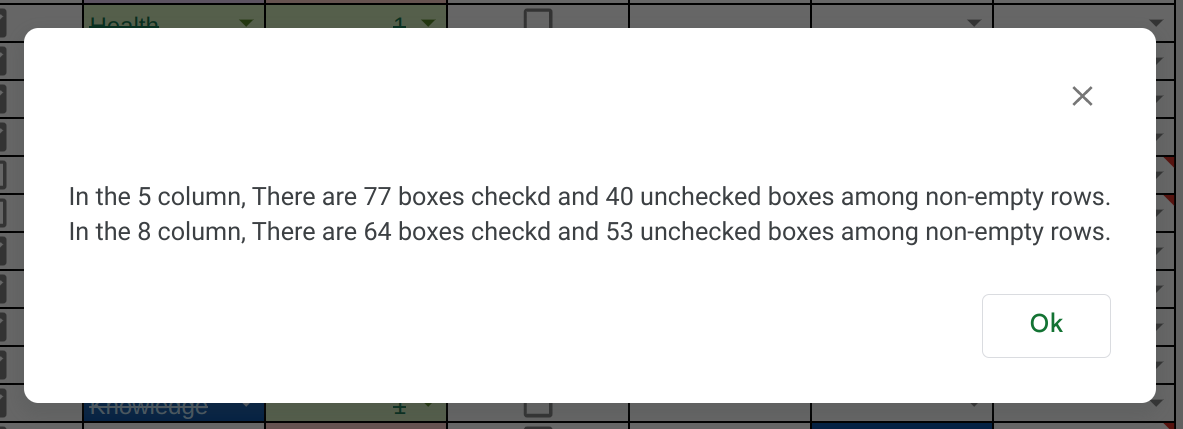# Using Apps Script to Count Checkboxes in Google Sheets

You have checkboxes in your spreadsheet, you’ll like to count them easily. Well, this script I’ll provide will help you with exactly that. Non-coders can easily follow instructions and add this script to their projects.

Namaste! This is Nibesh Khadka. I am a freelancer that develops scripts to automate Google Workspace Apps like Gmail, Google Sheets, Google Docs, etc.

## For Non-Coders

If you’re not a coder and don’t wanna be bothered with a tedious explanation then you can just follow these steps:

1. Open Script editor. On your spreadsheet click the extensions tab and open the apps script as shown in the image above.

2. Remove all the code in code.gs. Then, copy and paste the code from the Full Code section of this blog.

3. Save and then reload the spreadsheet. Now, reopen the Apps script as instructed in step 1 (Sometimes the script doesn't work without saving and reloading).

After this, you must make a minor edit as instructed below.

## Full Code

Here’s the full script for this blog:

``````// make minor change here.
// insert the number equivalent of your column with checkboxes here inside square boxes, like [1,2]
// for instance 1 for A, 5 for E, and so on.
const checkBoxColNumber = [5, 8];

/**
*/

}

/**
*/
function onOpen(e) {
}

/**
* CountCheckbox goes through the active sheet and counts the number of checked and unchecked box among non-empty rows
*/
function countCheckbox() {

// get sheet and data
let data = sheet.getDataRange().getValues();
data.shift();

// initate counts as zero
let trueCount = 0;
let falseCount = 0;

// value count will be a nested list but as same length as Counting Checkboxes
let checkBoxColValueCounts = [...checkBoxColNumber];

// loop through checkBoxColNumber list and data list
for (let j = 0; j < checkBoxColNumber.length; j++) {
for (let i = 0; i < data.length; i++) {
// [...new Set(data[i])].filter(String) check to get unique value if its either just true or false
if ([...new Set(data[i])].filter(String) === true || [...new Set(data[i])].filter(String) === false || data[i].join("") === "") { continue; }
// if values is true push increase true count else false count
if (data[i][checkBoxColNumber[j] - 1] === true) {
trueCount += 1;

} else {
falseCount += 1;

}
}

// add true and false count as nested list in respective place
checkBoxColValueCounts[j] = [trueCount, falseCount];
// reset
//console.log(checkBoxColValueCounts)
trueCount = 0;
falseCount = 0;
}

// create alert string with all info embeded
let countStatsString = "";
for (let j = 0; j < checkBoxColValueCounts.length; j++) {
countStatsString += `\n In the \${checkBoxColNumber[j]} column, There are \${checkBoxColValueCounts[j]} boxes checkd and \${checkBoxColValueCounts[j]} unchecked boxes among non-empty rows.`
}
}
``````

For this script to work in your spreadsheet you’re gonna have to make a tiny change in code.

``````// make minor change here.
// insert the number equivalent of your column with checkboxes here inside square boxes, like [1,2]
// for instance 1 for A, 5 for E, and so on.
const checkBoxColNumber = [5, 8];
``````

See this code in the beginning, you’ll have to replace [5, 8] these values with the number equivalent of the letters representing the columns with the checkbox values in your spreadsheet. For instance, 5 for E and 8 for H. If it’s just one column then insert one value inside the square box without the comma like .When you run this function you’ll see an alert box with the sum of all checked and unchecked boxes for non-empty rows in your spreadsheet.## Thank You for Your Time

If you would like me to write short and quick blogs like these then leave requests in the comments.

I make Add-Ons for Google and can also write Google Apps Scripts for you. If you need my services let me know.